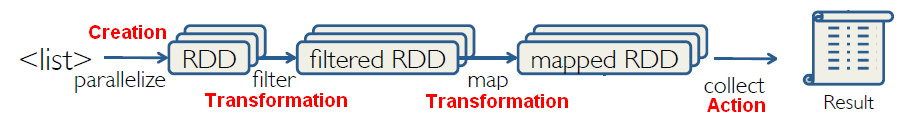# Spark - (Take|TakeOrdered)

The take action returns an array of the first n elements (not ordered) whereas takeordered returns an array with the first n elements after a sort

It's a Top N function

## Take

````take(n)`
```

Python:

``````rdd = sc.parallelize([1, 2, 3])
rdd.take(2)```
```
``````Value: [1,2] # as list
```
```

## Takeordered

````takeOrdered(n,key=func)`
```

Takeordered is an action that returns n elements ordered in ascending order as specified by the optional key function:

If key function returns a negative value (-1), the order is a descending order.

Python List:

``````rdd = sc.parallelize([5,3,1,2])
rdd.takeOrdered(3,lambda s: ‐1 * s)```
```
``````Value: [5,3,2] # as list
```
```

Python Tuple:

````topTenErrURLs = endpointSum.takeOrdered(10,lambda (x,y): -1*y)`
```

Discover MoreSpark - Action

in RDD. Reduce aggregates a data set element using a function. Takeordered and take returns n elements ordered or not Collect returns all of the elements of the RDD as an arraySpark - Sort

See also: takeOrdered sortByKey() return a new dataset (K, V) pairs sorted by keys in ascending order Syntax:...Spark RDD - String

Add the line number as a value of a tuple ? where: